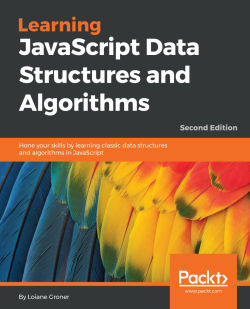### Learning JavaScript Data Structures and Algorithms - Second Edition4.1 (7 reviews total)
By Loiane Groner
• \$5 for 5 months Subscribe Access now
• \$35.99 eBook
Advance your knowledge in tech with a Packt subscription

• Instant online access to over 7,500+ books and videos
• Constantly updated with 100+ new titles each month
• Breadth and depth in over 1,000+ technologies

#### About this book

This book begins by covering basics of the JavaScript language and introducing ECMAScript 7, before gradually moving on to the current implementations of ECMAScript 6. You will gain an in-depth knowledge of how hash tables and set data structure functions, as well as how trees and hash maps can be used to search files in a HD or represent a database. This book is an accessible route deeper into JavaScript. Graphs being one of the most complex data structures you’ll encounter, we’ll also give you a better understanding of why and how graphs are largely used in GPS navigation systems in social networks.

Toward the end of the book, you’ll discover how all the theories presented by this book can be applied in real-world solutions while working on your own computer networks and Facebook searches.

Publication date:
June 2016
Publisher
Packt
Pages
314
ISBN
9781785285493

## Chapter 1. JavaScript—A Quick Overview

JavaScript is a very powerful language. It is the most popular language in the world and is one of the most prominent languages on the Internet. For example, GitHub (the world's largest code host, available at https://github.com ) hosts over 400,000 JavaScript repositories (the largest number of projects is in JavaScript; refer to http://goo.gl/ZFx6mg ). The number of projects in JavaScript in GitHub grows every year.

JavaScript is not a language that can only be used on the frontend. It can also be used in the backend, and Node.js is the technology responsible for this. The number of Node Package modules (https://www.npmjs.org/) also grows exponentially.

JavaScript is a must-have on your résumé if you are or will become a web developer.

In this chapter, you will learn the syntax and some necessary basic functionalities of Javascript, so we can start developing our own data structure and algorithms. We will cover:

• Setting up the environment

• Javascript basics

• Control structures

• Functions

• Object-oriented programming in Javascript

• Debugging and tools

• Introduction to ECMAScript 6 and ECMAScript 7

## JavaScript data structure and algorithms

In this book, you will learn about the most-used data structures and algorithms. However, why use JavaScript to learn about data structures and algorithms? We have already answered this question. JavaScript is very popular and is appropriate to learn about data structures because it is a functional language. Also, this can be a very fun way of learning something new as it is very different from (and easier than) learning about data structures with a standard language such as C or Java. And who said data structures and algorithms were only made for languages such as C and Java? You might need to implement some of these languages while developing for the frontend as well.

Learning about data structures and algorithms is very important. The first reason is that data structures and algorithms can solve the most common problems efficiently. This will make a difference to the quality of the source code you write in the future (including performance; if you choose the incorrect data structure or algorithm, depending on the scenario, you may have some performance issues). Secondly, algorithms are studied in college together with the introductory concepts of computer science. And thirdly, if you are planning to get a job in the greatest IT (Information Technology) companies (such as Google, Amazon, Ebay, and so on), data structures and algorithms are the subjects of interview questions.

Let's get the fun started!

## Setting up the environment

One of the pros of the JavaScript language compared to other languages is that you do not need to install or configure a complicated environment to get started with it. Every computer has the required environment already, even though the user may never write a single line of source code. All we need is a browser!

To execute the examples in this book, it is recommended that you have Google Chrome or Firefox installed (you can use the one you like the most), an editor of your preference (such as Sublime Text), and a web server (XAMPP or any other of your preference, but this step is optional). Chrome, Firefox, Sublime Text, and XAMPP are available for Windows, Linux, and Mac OS.

If you use Firefox, it is also recommended to install the Firebug add-on ( https://getfirebug.com/ ). We will present you with three options to set up your environment.

### The minimum setup to work with JavaScript

The simplest environment that you can use is a browser.

You can use Firefox along with Firebug. When you have Firebug installed, you will see the following icon in the upper-right corner:When you open Firebug (simply click on its icon), you will see the Console tab, and you will be able to write all your JavaScript code in its command-line area, as demonstrated in the following screenshot (to execute the source code, you need to click on the Run button):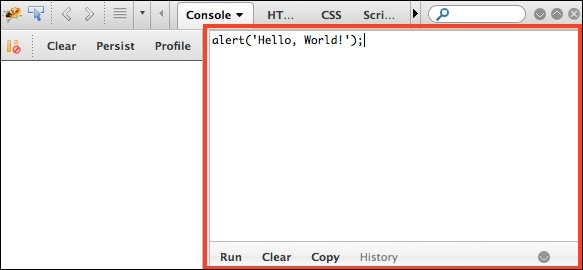You can also expand the command line to fit the entire available area of the Firebug add-on.

You can also use Google Chrome. Chrome already comes with Google Developer Tools. To open it, locate the setting and control icon and navigate to Tools | Developer Tools, as shown in the following screenshot: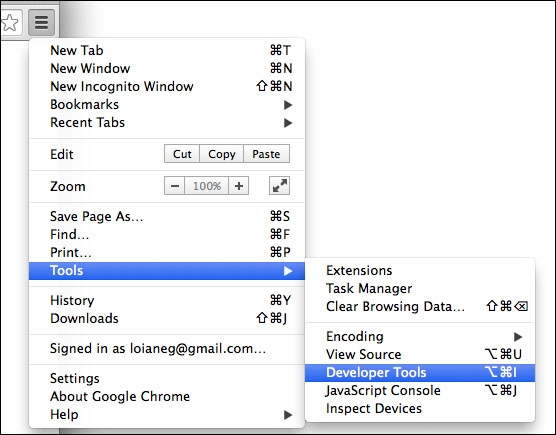Then, in the Console tab, you can write your own JavaScript code for testing, as follows: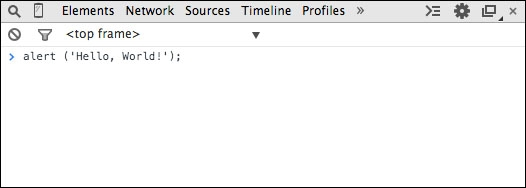### Using web servers (XAMPP)

The second environment you might want to install on your computer is also simple, but it's a little bit more complex than just using a browser.

You will need to install XAMPP ( https://www.apachefriends.org ) or any web server of your preference. Then, inside the XAMPP installation folder, you will find the `htdocs` directory. You can create a new folder in which you can execute the source code we will implement in this book, or you can download the source code from this book and extract it to the `htdocs` directory, as follows: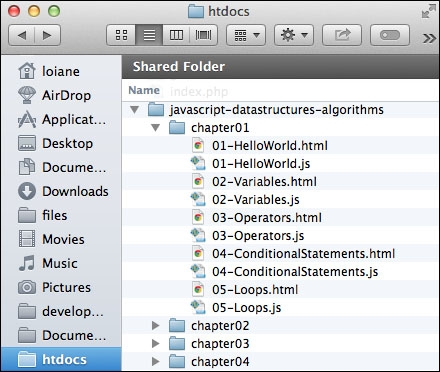Then, you can access the source code from your browser using your localhost URL (after starting the XAMPP server), as shown in the following screenshot (do not forget to enable Firebug or Google Developer Tools to see the output):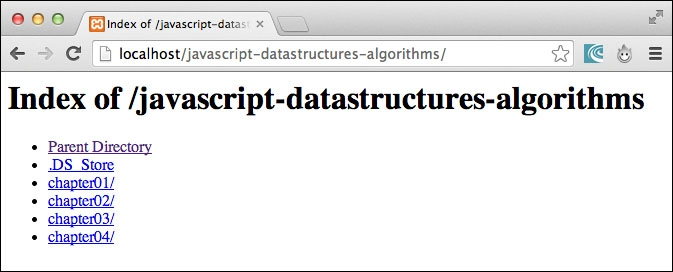### Tip

When executing the examples, always remember to have Google Developer Tools or Firebug open to see the output.

### It's all about JavaScript (Node.js)

The third option is having an environment that is 100 percent JavaScript! Instead of using XAMPP, which is an Apache server, we can use a JavaScript server.

To do so, we need to have Node.js installed. Go to http://nodejs.org/ and download and install Node.js. After this, open the terminal application (if you are using Windows, open the command prompt with Node.js, which was installed with Node.js) and run the following command:

```npm install http-server -g
```

Make sure you type the command and don't copy and paste it. Copying the command might give you some errors.

You can also execute the command as an administrator. For Linux and Mac systems, use the following command:

```sudo npm install http-server -g
```

This command will install `http-server`, which is a JavaScript server. To start a server and run the examples from this book in the terminal application, change the directory to the folder that contains the book's source code and type `http-server`, as displayed in the following screenshot: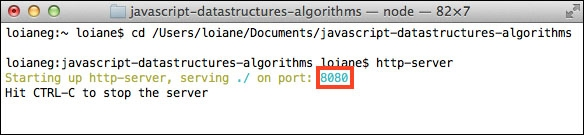To execute the examples, open the browser and access the localhost on the port specified by the `http-server` command, as follows: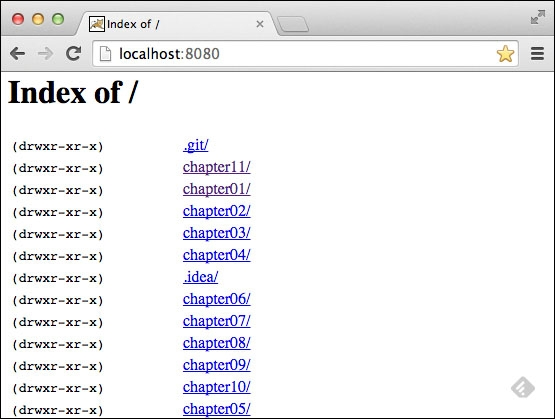### Tip

Detailed steps to download the code bundle are mentioned in the Preface of this book. Please have a look. The code bundle for the book is also hosted on GitHub at https://github.com/loiane/javascript-datastructures-algorithms. We also have other code bundles from our rich catalog of books and videos available at https://github.com/PacktPublishing/ . Check them out!

## JavaScript basics

Before we start diving into the various data structures and algorithms, let's have a quick overview of the JavaScript language. This section will present the JavaScript basics required to implement the algorithms we will create in the subsequent chapters.

To start, let's take a look at the two different ways we can use JavaScript code on an HTML page:

```<!DOCTYPE html>
<html>
<head>
<meta charset="UTF-8">
</head>
<body>
<script>
alert('Hello, World!');
</script>
</body>
</html>
```

The first way is demonstrated by the previous code. We need to create an HTML file and write this code on it. In this example, we are declaring the `script` tag inside the HTML file and, inside the `script` tag, we have the JavaScript code.

For the second example, we need to create a JavaScript file (we can save it as `01-HelloWorld.js`) and, inside this file, we will insert the following code:

```alert('Hello, World!');
```

Then, our HTML file will look similar to this:

```<!DOCTYPE html>
<html>
<head>
<meta charset="UTF-8">
</head>
<body>
<script src="01-HelloWorld.js">
</script>
</body>
</html>
```

The second example demonstrates how to include a JavaScript file inside an HTML file.

By executing any of these two examples, the output will be the same. However, the second example is best practice.

### Note

You may find JavaScript `include` statements or JavaScript code inside the `head` tag in some examples on the Internet. As best practice, we will include any JavaScript code at the end of the `body` tag. This way, the HTML will be parsed by the browser and displayed before the scripts are loaded. This boosts the performance of the page.

### Variables

Variables store data that can be set, updated, and retrieved whenever needed. Values that are assigned to a variable belong to a type. In JavaScript, the available types are numbers, strings, Booleans, functions, and objects. We also have undefined and null, along with arrays, dates, and regular expressions.

### Note

Although JavaScript has different available variable types, it is not a strongly typed language such as C/C++, C#, Java. In strongly typed languages, we need to declare the type of the variable along with its declaration (in Java, for example, to declare an integer variable, we use `int num = 1;`). In JavaScript, we only need to use the keyword `var`, and we do not need to declare the variable type. For this reason, JavaScript is not a strongly typed language.

The following is an example of how to use variables in JavaScript:

```var num = 1; //{1}
num = 3; //{2}
var price = 1.5; //{3}
var name = 'Packt'; //{4}
var trueValue = true; //{5}
var nullVar = null; //{6}
var und;  //{7}
```
• On line `{1}`, we have an example of how to declare a variable in JavaScript (we are declaring a number). Although it is not necessary to use the `var` keyword declaration, it is good practice to always specify when we declare a new variable.

• On line `{2}`, we updated an existing variable. JavaScript is not a strongly typed language. This means you can declare a variable, initialize it with a number, and then update it with a string or any other datatype. Assigning a value to a variable that is different from its original type is also not good practice.

• On line `{3}`, we also declared a number, but this time it is a decimal floating point. On line `{4}`, we declared a string; on line `{5}`, we declared a Boolean. On line `{6}`, we declared a `null` value, and on line `{7}`, we declared an undefined variable. A `null` value means no value, and `undefined` means a variable that has been declared but not yet assigned a value. Take a look at the following:

```        console.log("num: "+ num);
console.log("name: "+ name);
console.log("trueValue: "+ trueValue);
console.log("price: "+ price);
console.log("nullVar: "+ nullVar);
console.log("und: "+ und);
```

If we want to see the value of each variable we declared, we can use `console.log` to do so, as listed in the previous code snippet.

### Note

We have three ways of outputting values in JavaScript that we can use with the examples of this book. The first one is `alert('My text here')`, which outputs an alert window on the browser, and the second one is `console.log('My text here')`, which outputs text on the Console tab of the debug tool (Google Developer Tools or Firebug, depending on the browser you are using). The third way is outputting the value directly on the HTML page that is rendered by the browser using `document.write('My text here')`. You can use the option that you feel most comfortable with.

The `console.log` method also accepts more than just arguments. Instead of `console.log("num: "+ num)`, we can also use `console.log("num: ", num)`.

We will discuss functions and objects later in this chapter.

#### Variable scope

Scope refers to where in the algorithm we can access the variable (it can also be a function when we work with function scopes). There are local and global variables.

Let's look at an example:

```var myVariable = 'global';
myOtherVariable = 'global';

function myFunction(){
var myVariable = 'local';
return myVariable;
}

function myOtherFunction(){
myOtherVariable = 'local';
return myOtherVariable;
}

console.log(myVariable);   //{1}
console.log(myFunction()); //{2}

console.log(myOtherVariable);   //{3}
console.log(myOtherFunction()); //{4}
console.log(myOtherVariable);   //{5}
```
• Line `{1}` will output `global` because we are referring to a `global` variable.

• Line `{2}` will output `local` because we declared the `myVariable` variable inside the `myFunction` function as a local variable, so the scope will only be inside `myFunction`.

• Line `{3}` will output `global` because we are referencing the global variable named `myOtherVariable` that was initialized on the second line of the example.

• Line `{4}` will output `local`. Inside the `myOtherFunction` function, we  referencing the `myOtherVariable` global variable and assigning the value `local` to it because we are not declaring the variable using the `var` keyword.

• For this reason, line `{5}` will output `local` (because we changed the value of the variable inside `myOtherFunction`).

You may hear that global variables in JavaScript are evil and this is true. Usually, the quality of JavaScript source code is measured by the number of global variables and functions (a large number is bad). So, whenever possible, try avoiding global variables.

### Operators

We need operators when performing any operation in a programming language. JavaScript also has arithmetic, assignment, comparison, logical, bitwise, and unary operators, among others. Let's take a look at these:

```var num = 0; // {1}
num = num + 2;
num = num * 3;
num = num / 2;
num++;
num--;

num += 1; // {2}
num -= 2;
num *= 3;
num /= 2;
num %= 3;

console.log('num == 1 : ' + (num == 1)); // {3}
console.log('num === 1 : ' + (num === 1));
console.log('num != 1 : ' + (num != 1));
console.log('num > 1 : ' + (num > 1));
console.log('num < 1 : ' + (num < 1));
console.log('num >= 1 : ' + (num >= 1));
console.log('num <= 1 : ' + (num <= 1));

console.log('true && false : ' + (true && false)); // {4}
console.log('true || false : ' + (true || false));
console.log('!true : ' + (!true));
```

On line `{1}`, we have the arithmetic operators. In the following table, we have the operators and their descriptions:

 Arithmetic operator Description `+` Addition `-` Subtraction `*` Multiplication `/` Division `%` Modulus (remainder of a division operation) `++` Increment `--` Decrement

On line `{2}`, we have the assignment operators. In the following table, we have the operators and their descriptions:

 Assignment operator Description `=` Assignment `+=` Addition assignment (x += y) == (x = x + y) `-=` Subtraction assignment (x -= y) == (x = x - y) `*=` Multiplication assignment (x *= y) == (x = x * y) `/=` Division assignment (x /= y) == (x = x / y) `%=` Remainder assignment (x %= y) == (x = x % y)

On line `{3}`, we have the comparison operators. In the following table, we have the operators and their descriptions:

 Comparison operator Description `==` Equal to `===` Equal to (value and object type both) `!=` Not equal to `>` Greater than `>=` Greater than or equal to `<` Less than `<=` Less than or equal to

Finally, on line `{4}`, we have the logical operators. In the following table, we have the operators and their descriptions:

 Logical operator Description `&&` And `||` Or `!` Not

JavaScript also supports bitwise operators, which are shown as follows:

```console.log('5 & 1:', (5 & 1));
console.log('5 | 1:', (5 | 1));
console.log('~ 5:', (~5));
console.log('5 ^ 1:', (5 ^ 1));
console.log('5 << 1:', (5 << 1));
console.log('5 >> 1:', (5 >> 1));
```

The following table contains a more detailed description of the bitwise operators:

 Bitwise operator Description `&` And `|` Or `~` Not `^` Xor `<<` Left shift `>>` Right shift

The `typeof` operator returns the type of the variable or expression. For example, have a look at the following code:

```console.log('typeof num:', typeof num);
console.log('typeof Packt:', typeof 'Packt');
console.log('typeof true:', typeof true);
console.log('typeof [1,2,3]:', typeof [1,2,3]);
console.log('typeof {name:John}:', typeof {name:'John'});
```

The output will be as follows:

```typeof num: number
typeof Packt: string
typeof true: boolean
typeof [1,2,3]: object
typeof {name:John}: object
```

JavaScript also supports the `delete` operator, which deletes a property from an object. Take a look at the following code:

```var myObj = {name: 'John', age: 21};
delete myObj.age;
console.log(myObj); //outputs Object {name: "John"}
```

In this book's algorithms, we will use some of these operators.

### Truthy and falsy

In JavaScript, true and false are a little bit tricky. In most languages, the Boolean values `true` and `false` represent the true/false results. In JavaScript, a string such as "Packt" has the value true, for example.

The following table can help us better understand how true and false work in JavaScript:

 Value type Result undefined `false` null `false` Boolean true is `true` and false is `false` Number The result is `false` for `+0`, `-0`, or `NaN`; otherwise, the result is `true` String The result is `false` if the string is empty (length is 0); otherwise, the result is `true` (length > 1) Object `true`

Let's consider some examples and verify their output:

```function testTruthy(val){
return val ? console.log('truthy') : console.log('falsy');
}

testTruthy(true); //true
testTruthy(false); //false
testTruthy(new Boolean(false)); //true (object is always true)

testTruthy(''); //false
testTruthy('Packt'); //true
testTruthy(new String('')); //true (object is always true)

testTruthy(1); //true
testTruthy(-1); //true
testTruthy(NaN); //false
testTruthy(new Number(NaN)); //true (object is always true)

testTruthy({}); //true (object is always true)

var obj = {name:'John'};
testTruthy(obj); //true
testTruthy(obj.name); //true
testTruthy(obj.age); //false (age does not exist)
```

### Functions of the equals operators (== and ===)

The two equal operators supported by JavaScript can cause a little bit of confusion when working with them.

When using `==`, values can be considered equal even when they are of different types. This can be confusing even for a senior JavaScript developer. Let's analyze how `==` works using the following table:

 Type(x) Type(y) Result null undefined `true` undefined null `true` Number String `x == toNumber(y)` String Number `toNumber(x) == y` Boolean Any `toNumber(x) == y` Any Boolean `x == toNumber(y)` String or Number Object `x == toPrimitive(y)` Object String or Number `toPrimitive(x) == y`

If x and y are of the same type, then JavaScript will use the `equals` method to compare the two values or objects. Any other combination that is not listed in the table gives a false result.

The `toNumber` and `toPrimitive` methods are internal and evaluate the values according to the tables that follow.

The `toNumber` method is presented here:

 Value type Result undefined This is` NaN`. null This is` +0`. Boolean If the value is `true`, the result is `1`; if the value is `false`, the result is `+0`. Number This is the value of the number. String This parses the string into a number. If the string consists of alphabetical characters, the result is `NaN`; if the string consists of numbers, it is transformed into a number. Object This is` toNumber(toPrimitive(value))`.

Finally, `toPrimitive` is presented here:

 Value type Result Object If `valueOf` returns a primitive value, this returns the primitive value; otherwise, if `toString` returns a primitive value, this returns the primitive value and otherwise returns an error.

Let's verify the results of some examples. First, we know that the output of the following code is `true` (string length > 1):

```console.log('packt' ? true : false);
```

Now, what about the following code? Let's take a look:

```console.log('packt' == true);
```

The output is `false`, so let's understand why:

• First, it converts the Boolean value using `toNumber`, so we have `packt == 1`.

• Then, it converts the string value using `toNumber`. As the string consists of alphabetical characters, it returns `NaN`, so we have `NaN == 1`, which is false.

What about the following code? Let's take a look:

```console.log('packt' == false);
```

The output is also `false`, and the following are the steps:

• First, it converts the Boolean value using `toNumber`, so we have `packt == 0`.

• Then, it converts the string value using `toNumber`. As the string consists of alphabetical characters, it returns `NaN`, so we have `NaN == 0`, which is false.

What about the `=== `operator? It is much easier. If we are comparing two values of different types, the result is always false. If they have the same type, they are compared according to the following table:

 Type(x) Values Result Number x has the same value as y (but not `NaN`) `true` String x and y are identical characters `true` Boolean x and y are both `true` or both `false` `true` Object x and y reference the same object `true`

If x and y are different types, then the result is false.

Let's consider some examples:

```console.log('packt' === true); //false

console.log('packt' === 'packt'); //true

var person1 = {name:'John'};
var person2 = {name:'John'};
console.log(person1 === person2); //false, different objects
```

## Control structures

JavaScript has a similar set of control structures as the C and Java languages. Conditional statements are supported by `if...else` and `switch`. Loops are supported by the `while`, `do...while`, and `for` constructs.

### Conditional statements

The first conditional statement we will take a look at is the `if...else` construct. There are a few ways we can use the `if...else` construct.

We can use the `if` statement if we want to execute a script only if the condition is `true`, as follows:

```var num = 1;
if (num === 1) {
console.log("num is equal to 1");
}
```

We can use the `if...else` statement if we want to execute a script and the condition is `true` or another script just in case the condition is `false` (`else`), as follows:

```var num = 0;
if (num === 1) {
console.log("num is equal to 1");
} else {
console.log("num is not equal to 1, the value of num is " + num);
}
```

The `if...else` statement can also be represented by a ternary operator. For example, take a look at the following `if...else` statement:

```if (num === 1){
num--;
} else {
num++;
}
```

It can also be represented as follows:

```(num === 1) ? num-- : num++;
```

Also, if we have several scripts, we can use `if...else` several times to execute different scripts based on different conditions, as follows:

```var month = 5;
if (month === 1) {
console.log("January");
} else if (month === 2){
console.log("February");
} else if (month === 3){
console.log("March");
} else {
console.log("Month is not January, February or March");
}
```

Finally, we have the `switch` statement. If the condition we are evaluating is the same as the previous one (however, it is being compared to different values), we can use the `switch` statement:

```var month = 5;
switch(month) {
case 1:
console.log("January");
break;
case 2:
console.log("February");
break;
case 3:
console.log("March");
break;
default:
console.log("Month is not January, February or March");
}
```

One thing that is very important in a `switch` statement is the usage of the `case` and `break` keywords. The `case` clause determines whether the value of `switch` is equal to the value of the `case` clause. The `break` statement stops the `switch` statement from executing the rest of the statement (otherwise, it will execute all the scripts from all `case` clauses below the matched case until a `break` statement is found in one of the `case` clauses). Finally, we have the `default` statement, which is executed by default if none of the case statements are `true` (or if the executed `case` statement does not have the `break` statement).

### Loops

Loops are very often used when we work with arrays (which are the subject of the next chapter). Specifically, we will use the `for` loop in our algorithms.

The `for` loop is exactly the same as in C and Java. It consists of a loop counter that is usually assigned a numeric value, then the variable is compared against another value (the script inside the `for` loop is executed while this condition is true), and finally the numeric value is increased or decreased.

• In the following example, we have a `for` loop. It outputs the value of `i` on the console, while `i` is less than `10`; `i` is initiated with `0`, so the following code will output the values 0 to 9:

```        for (var i=0; i<10; i++) {
console.log(i);
}
```
• The next loop construct we will look at is the `while` loop. The script inside the `while` loop is executed while the condition is true. In the following code, we have a variable, `i`, initiated with the value `0`, and we want the value of `i` to be output while `i` is less than 10 (or less than or equal to 9). The output will be the values from 0 to 9:

```        var i = 0;
while(i<10)
{
console.log(i);
i++;
}
```
• The `do...while` loop is very similar to the `while` loop. The only difference is that in the `while` loop, the condition is evaluated before executing the script, and in the `do...while` loop, the condition is evaluated after the script is executed. The `do...while` loop ensures that the script is executed at least once. The following code also outputs the values from 0 to 9:

```        var i = 0;
do {
console.log(i);
i++;
} while (i<10)
```

## Functions

Functions are very important when working with JavaScript. We will also use functions a lot in our examples.

The following code demonstrates the basic syntax of a function. It does not have arguments or the `return` statement:

```function sayHello() {
console.log('Hello!');
}
```

To call this code, we simply use the following call:

```sayHello();
```

We can also pass arguments to a function. Arguments are variables with which a function is supposed to do something. The following code demonstrates how to use arguments with functions:

```function output(text) {
console.log(text);
}
```

To use this function, we can use the following code:

```output('Hello!');
```

You can use as many arguments as you like, as follows:

```output('Hello!', 'Other text');
```

In this case, only the first argument is used by the function and the second one is ignored.

A function can also return a value, as follows:

```function sum(num1, num2) {
return num1 + num2;
}
```

This function calculates the sum of two given numbers and returns its result. We can use it as follows:

```var result = sum(1,2);
output(result);
```

## Object-oriented programming in Javascript

JavaScript objects are very simple collections of name-value pairs. There are two ways of creating a simple object in JavaScript. The first way is as follows:

```var obj = new Object();
```

And the second way is as follows:

```var obj = {};
```

We can also create an object entirely, as follows:

```obj = {
name: {
first: 'Gandalf',
last: 'the Grey'
},
address: 'Middle Earth'
};
```

As we can see, to declare a JavaScript object, [key, value] pairs are used, where the key can be considered an attribute of the object and the value is the attribute value. All classes that we will create in this book are JavaScript objects, such as `Stack`, `Set`, `LinkedList`, `Dictionary`, `Tree`, `Graph`, and so on.

In object-oriented programming (OOP), an object is an instance of a class. A class defines the characteristics of the object. For our algorithms and data structures, we will create some classes that will represent them. This is how we can declare a class that represents a book:

```function Book(title, pages, isbn){
this.title = title;
this.pages = pages;
this.isbn = isbn;
}
```

To instantiate this class, we can use the following code:

```var book = new Book('title', 'pag',  'isbn');
```

Then, we can access its attributes and update them as follows:

```console.log(book.title); //outputs the book title
book.title = 'new title'; //updates the value of the book title
console.log(book.title); //outputs the updated value
```

A class can also contain functions. We can declare and use a function as the following code demonstrates:

```Book.prototype.printTitle = function(){
console.log(this.title);
};
book.printTitle();
```

We can declare functions directly inside the class definition as well:

```function Book(title, pages, isbn){
this.title = title;
this.pages = pages;
this.isbn = isbn;
this.printIsbn = function(){
console.log(this.isbn);
}
}
book.printIsbn();

```

### Note

In the prototype example, the `printTitle` function will be shared between all the instances and only one copy will be created. When we use a class-based definition, as in the previous example, each instance will have its own copy of the functions. Using the prototype method saves memory and processing cost with regard to assigning the functions to the instance. However, you can only declare public functions and properties using the prototype method. With a class-based definition, you can declare private functions and properties and the other methods inside the class can also access them. ECMAScript 6 introduces a simplified syntax very similar to the class-based example and it is prototype based. We will discuss more on this later in this chapter.

## Debugging and tools

Knowing how to program with JavaScript is important, but so is knowing how to debug your code. Debugging is very useful in helping you find bugs in your code, but it can also help you execute your code at a lower speed so that you can see everything that is happening (the stack of methods called, variable assignment, and so on). It is highly recommended that you spend some time debugging the source code of this book to see every step of the algorithm (it might help you understand it better as well).

Both Firefox and Chrome support debugging. A great tutorial from Google that shows you how to use Google Developer Tools to debug JavaScript can be found at https://developer.chrome.com/devtools/docs/javascript-debugging .

You can use any text editor of your preference. However, there are other great tools that can help you be more productive when working with JavaScript as well, which are as follows:

• Aptana: This is a free and open source IDE that supports JavaScript, CSS3, and HTML5, among other languages ( http://www.aptana.com/ ).

• WebStorm: This is a very powerful JavaScript IDE with support for the latest web technologies and frameworks. It is a paid IDE, but you can download a 30-day trial version ( http://www.jetbrains.com/webstorm/ ).

• Sublime Text: This is a lightweight text editor and you can customize it by installing plugins. You can buy the license to support the development team, but you can also use it for free (the trial version does not expire) at http://www.sublimetext.com/ .

• Atom: This is also a free lightweight text editor created by GitHub. It has great support for JavaScript and it can also be customized by installing plugins ( https://atom.io/ ).

## Introducing ECMAScript

If you follow the news and latest trends about JavaScript, you might have heard the hype about ECMAScript 6 and ECMAScript 7. What does ECMAScript have to do with JavaScript and is there a difference?

ECMAScript is a scripting language specification. JavaScript is an implementation of this specification, as are Jscript and ActionScript.

### ECMAScript 6 and ECMAScript 7

As we know, JavaScript is a language that runs mostly on browsers (also in servers using NodeJS), and each browser can implement its own version of the available functionalities of JavaScript (as you will learn later on in this book). This specific implementation is based on ECMAScript. Thus, the browsers offer mostly the same functionalities (our JavaScript code will run in all browsers); however, each functionality's behavior may be a little bit different from browser to browser.

All the code presented in this chapter so far is based on ECMAScript 5, which became a standard in December 2009. The most recent release of ECMAScript, at the time this book is being written, is ECMAScript 6, which was standardized in July 2015, almost 6 years after its previous version. The committee responsible for drafting the ECMAScript specifications made the decision to move to a yearly model to define new standards, where new features would be added as they were approved. For this reason, ECMAScript sixth edition was renamed to ECMAScript 2015 (ES6). There is a new version that is also being prepared to be released in the summer of 2016, which is called ECMAScript 2016 or ECMAScript 7 (ES7).

In this topic, we will cover some of the new functionalities introduced in ES6 and ES7.

### The compatibility table

It is important to know that, even though ES6 has already been released, its features might not be supported by all browsers. For a better experience, it is best to use the latest version available for the browser you choose to use (Firefox or Chrome).

At the following links, you can verify which features are available in each browser:

Even if the features are not yet available, we can start using new syntax and new functionalities today.

By default, Firefox adds support to ES6 and ES7 as their team ships the implementation of the functionalities.

In Google Chrome, you can enable the functionalities by enabling the experimental JavaScript flag by opening the URL ``` chrome://flags ```, as demonstrated in the following image: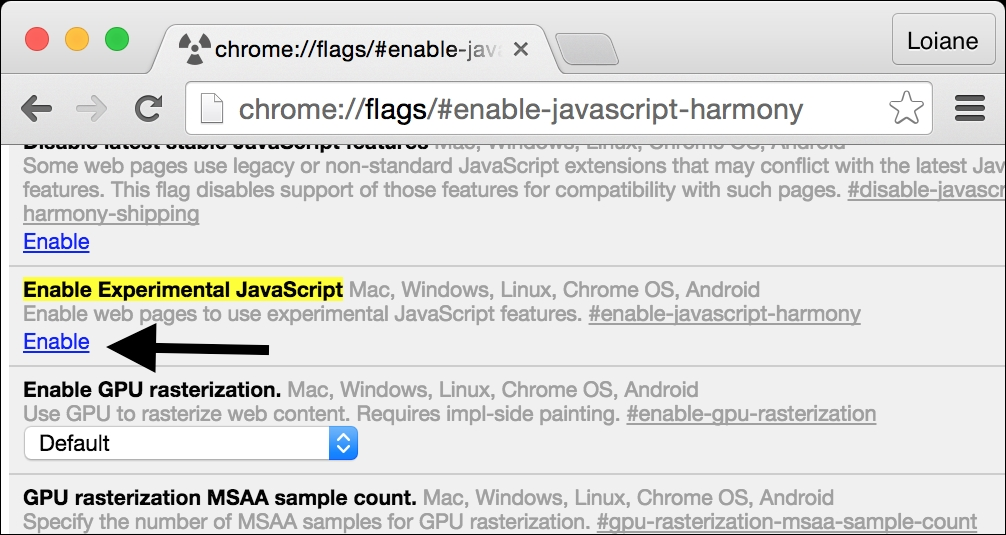### Note

Even with the Enable Experimental JavaScript flag enabled, some of the ES6 features might not be supported in Chrome. The same can be applied to Firefox. To know exactly which features are already supported in each browser, take a look at the compatibility table.

#### Using Babel.js

Babel ( https://babeljs.io ) is a JavaScript transpiler, also known as a source-to-source compiler. It converts JavaScript code with ES6 and ES7 language features to equivalent code that uses only language features from the widely supported ES5 specification.

There are many different ways of using Babel.js. One of them is installing it according to its setup (https://babeljs.io/docs/setup/ ). Another one is using it directly in the browser through its Try it out option ( https://babeljs.io/repl/ ), as demonstrated in the following screenshot: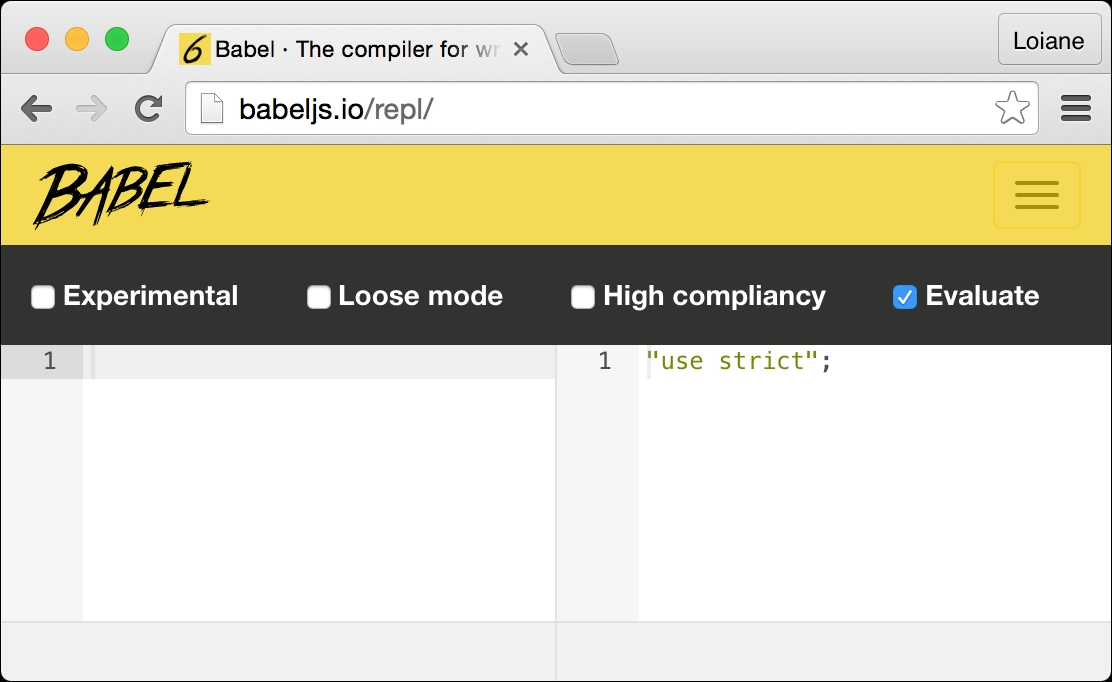Along with each example of ES6 and ES7 that will be presented in the following topics, we will also provide a link so that you can run and test the examples in Babel.

## ECMAScript 6 functionalities

In this topic, we will demonstrate how to use some of the new functionalities of ES6, which may be useful in the everyday JavaScript coding and which will also be useful to simplify the examples presented in the next chapters of this book. Among the functionalities, we will cover the following ones:

• `let` and `const`

• Template literals

• Destructuring

• Spread operator

• Arrow functions using `=>`

• Classes

### Declaring variables with let instead of var

Until ES5, we could declare variables in any place of our code, even if we overwrote the variables declaration, as in the following code:

```var framework = 'Angular';
var framework = 'React';
console.log(framework);
```

The output of the preceding code is `React` as the last variable declared, named framework, was assigned this value. In the previous code, we had two variables with the same name; this is very dangerous and might drive the code to an incorrect output.

Other languages, such as C, Java, and C#, do no allow this behavior. With ES6, a new keyword was introduced, called `let`. Let is the new `var `keyword, meaning we can simply substitute the keyword `var` for `let`. In the following code, we have an example:

```let language = 'JavaScript!'; //{1}
let language = 'Ruby!'; // {2} - throws error
console.log(language);
```

Line `{2}` will throw an error because a variable named language is already declared in the same scope (line `{1}`). We will discuss the `let` and variables scope in the next topic.

### Tip

The preceding code can be tested and executed at the URL https://goo.gl/he0udZ .

#### Variables scope with let

To understand how variables declared with `let` keyword work, let's use the following example (you can run the example using the URL  https://goo.gl/NbsVvg ):

```let movie = 'Lord of the Rings'; //{1}
//var movie = 'Batman v Superman'; //throws error, variable movie already declared

function starWarsFan(){
let movie = 'Star Wars'; //{2}
return movie;
}

function marvelFan(){
movie = 'The Avengers'; //{3}
return movie;
}

function blizzardFan(){
let isFan = true;
let phrase = 'Warcraft'; //{4}
console.log('Before if: ' + phrase);
if (isFan){
let phrase = 'initial text'; //{5}
phrase = 'For the Horde!';   //{6}
console.log('Inside if: ' + phrase);
}
phrase = 'For the Alliance!';    //{7}
console.log('After if: ' + phrase);
}

console.log(movie); //{8}
console.log(starWarsFan()); //{9}
console.log(marvelFan()); //{10}
console.log(movie);       //{11}
blizzardFan(); //{12}
```

This will be the output from the previous code:

```Lord of the Rings
Star Wars
The Avengers
The Avengers
Before if: Warcraft
Inside if: For the Horde!
After if: For the Alliance!
```

Now, we will discuss the reason we got this output.

• On line `{1}`, we declared a variable `movie` with the value `Lord of the Rings`, and we output its value on line `{8}`. This variable has global scope, as you learned in the Variable scope section of this chapter.

• On line `{9}`, we executed the `starWarsFan `function. Inside this function, we also declared a variable named `movie` on line `{2}`. The output from this function is `Star Wars` because the variable from line `{2}` has local scope, meaning it is only valid inside this function.

• On line `{10}`, we executed the `marvelFan `function. Inside this function, we changed the value of the `movie `variable (line `{3}`). This variable made a reference to the global variable declared on line `{1}`. This is why we got the output `The Avengers` on line `{10}` and also on line `{11}`, where we output the global variable.

• Finally, we executed the `blizzardFan` function on line `{12}`. Inside this function, we declared a variable named `phrase` (line `{4}`) with the scope of the function. Then, on line `{5}`, again, we will declare a variable named `phrase`, but this time, this variable will have only a scope inside the `if` statement.

• On line `{6}`, we changed the value of `phrase`. As we are still inside the `if` statement, only the variable declared on line `{5}` would have its value changed.

• Then, on line `{7}`, we again changed the value of `phrase`, but as we are not inside the block of the `if` statement, the value of the variable declared on line `{4}` is changed.

Again, this scope behavior is the same as in other programming languages, such as Java or C. However, this was only introduced in JavaScript through ES6.

### Constants

ES6 also introduced the keyword `const`. Its behavior is the same thing as the keyword `let`; the only difference is that a variable defined as `const` has a read-only value, meaning a constant value.

For example, consider the following code:

```const PI = 3.141593;
PI = 3.0; //throws error
console.log(PI);
```

When we try to assign a new value to `PI` or even try to redeclare it as `var PI` or `let PI`, the code will throw an error saying that `PI` is read only.

### Tip

The preceding code can be executed at https://goo.gl/4xuWrC .

### Template literals

Template literals are really nice because we can create strings without the need to concatenate the values.

For example, consider the following examples written with ES5:

```var book = {
name: 'Learning JavaScript DataStructures and Algorithms'
};
console.log('You are reading ' + book.name + '.,\n    and this is a new line\n    and so is this.');
```

We can improve the syntax of the previous `console.log` output with the following code:

```console.log(`You are reading \${book.name}.,
and this is a new line
and so is this.`);
```

Template literals are enclosed by backticks (```). To interpolate a variable value, we will simply set the variable value inside a dollar sign and curly braces (`\${}`), as we did with `book.name`.

Template literals can also be used for multiline strings. There is no need to use `\n` anymore. Simply hit Enter on the keyboard to take the string to a new line, as was done with `and this is a new line` in the previous example.

This functionality will be very useful in our examples to simplify the output!

### Tip

The preceding examples can be executed at https://goo.gl/PTqnwO .

### Arrow functions

Arrow functions are a great way of simplifying the syntax of functions in ES6. Consider the following example:

```var circleArea = function circleArea(r) {
var PI = 3.14;
var area = PI * r * r;
return area;
};
console.log(circleArea(2));
```

We can simplify the syntax of the preceding code to the following code:

```let circleArea = (r) => { //{1}
const PI = 3.14;
let area = PI * r * r;
return area;
}
console.log(circleArea(2));
```

The main difference is on line `{1}` of the example, on which we can omit the keyword function using `=>`.

If the function has a single statement, we can use a simpler version as well, by omitting the keyword `return`. Take a look at the following:

```let circleArea2 = (r) => 3.14 * r * r;
console.log(circleArea2(2));
```

### Tip

The preceding examples can be executed athttps://goo.gl/CigniJ .

### Default parameter values for functions

With ES6, it is also possible to define default parameter values for functions. The following is an example:

```function sum (x = 1, y = 2, z = 3) {
return x + y + z
};
console.log(sum(4,2)); //outputs 9
```

As we are not passing `z` as a parameter, it will have value 3 by default. So, `4 + 2 + 3 == 9`.

Before ES6, we would have to write the preceding function as the following code:

```function sum (x, y, z) {
if (x === undefined)
x = 1;
if (y === undefined)
y = 2;
if (z === undefined)
z = 3;
return x + y + z;
};
```

With ES6, we can save a few lines of code using the default parameter values functionality.

### Tip

The preceding example can be executed at https://goo.gl/2MiJ59 .

### Declaring the spread and rest operators

In ES5, we can turn arrays into parameters using the `apply() `function. ES6 has the spread operator (`...`) for this purpose. For example, consider the function sum we declared in the previous topic. We can execute the following code to pass the `x`, `y`, and `z` parameters:

```var params = [3, 4, 5];
console.log(sum(...params));
```

The preceding code is the same as the code written in ES5, as follows:

```var params = [3, 4, 5];
console.log(sum.apply(undefined, params));
```

The spread operator (`...`) can also be used as a rest parameter in functions to replace `arguments`. Consider the following example:

```function restParamaterFunction (x, y, ...a) {
return (x + y) * a.length;
}
console.log(restParamaterFunction(1, 2, "hello", true, 7)); //outputs 9;
```

The preceding code is the same as the following:

```function restParamaterFunction (x, y) {
var a = Array.prototype.slice.call(arguments, 2);
return (x + y) * a.length;
};
```

### Tip

The spread operator example can be executed at https://goo.gl/8equk5 , and the rest parameter example can be executed at https://goo.gl/LaJZqU .

#### Enhanced object properties

ES6 introduces a concept called array destructuring, which is a way of initializing variables at once. For example, consider the following:

```var [x, y] = ['a', 'b'];
```

Executing the preceding code is the same as doing the following:

```var x = 'a';
var y = 'b';
```

Array destructuring can also be performed to swap values at once without the need to create temp variables, as follows:

```[x, y] = [y, x];
```

The preceding code is the same as the following one:

```var temp = x;
x = y;
y = temp;
```

This will be very useful when you learn sorting algorithms as this swap values is very common.

There is also another functionality, called property shorthand, which is another way of performing the destructuring of objects. For example, consider the following example:

```var [x, y] = ['a', 'b'];
var obj = { x, y };
console.log(obj); // { x: "a", y: "b" }
```

The preceding code is the same as doing the following:

```var x = 'a';
var y = 'b';
var obj2 = { x: x, y: y };
console.log(obj2); // { x: "a", y: "b" }
```

The last functionality we will discuss in this topic is called the method property. This allows developers to declare functions inside objects as if they were properties. The following is an example:

```var hello = {
name : 'abcdef',
printHello(){
console.log('Hello');
}
}
console.log(hello.printHello());
```

The preceding code can also be written as follows:

```var hello = {
name: 'abcdef',
printHello: function printHello() {
console.log('Hello');
}
};
```

The three examples presented can be executed at:

### Object-oriented programming with classes

ES6 also introduced a cleaner way of declaring classes. You learned that we can declare a class named `Book` in the Object oriented programming section this way:

```function Book(title, pages, isbn){ //{1}
this.title = title;
this.pages = pages;
this.isbn = isbn;
}
Book.prototype.printTitle = function(){
console.log(this.title);
};
```

With ES6, we can simplify the syntax and use the following code:

```class Book { //{2}
constructor (title, pages, isbn) {
this.title = title;
this.pages = pages;
this.isbn = isbn;
}
printIsbn(){
console.log(this.isbn);
}
}
```

We can simply use the keyword `class` and declare a class with a `constructor` function and other functions as well—for example, the `printIsbn `function. The code for the class `Book` declared on line `{1}` has the same effect and output as the code declared on line `{2}`:

```let book = new Book('title', 'pag',  'isbn');
console.log(book.title); //outputs the book title
book.title = 'new title'; //update the value of the book title
console.log(book.title); //outputs the book title
```

### Tip

The preceding example can be executed at  https://goo.gl/UhK1n4 .

#### Inheritance

As we have a new way of declaring classes, there is also a simplified syntax to use inheritance between classes. Let's take a look at an example:

```class ITBook extends Book { //{1}
constructor (title, pages, isbn, technology) {
super(title, pages, isbn); //{2}
this.technology = technology;
}
printTechnology(){
console.log(this.technology);
}
}

let jsBook = new ITBook('Learning JS Algorithms', '200', '1234567890', 'JavaScript');
console.log(jsBook.title);
console.log(jsBook.printTechnology());
```

We can extend another class and inherit its behavior using the keyword `extends` (line `{1}`). Inside the constructor, we can also refer to the `constructor` superclass using the keyword `super` (line `{2}`).

Although the syntax of this new way of declaring classes in JavaScript is very similar to other programming languages such as Java and C/C++, it is good to remember that JavaScript object-oriented programming is done through a prototype.

### Tip

The preceding example can be executed at  https://goo.gl/hgQvo9 .

#### Working with getters and setters

With the new class syntax, it is also possible to create getter and setter functions for the class attributes. Although class attributes are not private as in other object-oriented languages (the encapsulation concept), it is good to follow a naming pattern.

The following is an example of a class declaring a `get` and `set` function along with its use:

```class Person {
constructor (name) {
this._name = name; //{1}
}
get name() { //{2}
return this._name;
}
set name(value) { //{3}
this._name = value;
}
}

let lotrChar = new Person('Frodo');
console.log(lotrChar.name); //{4}
lotrChar.name = 'Gandalf';    //{5}
console.log(lotrChar.name);
lotrChar._name = 'Sam'; //{6}
console.log(lotrChar.name);
```

To declare a `get` and `set` function, we simply need to use the keyword `get` or `set` in front of the function name (lines `{2}` and `{3}`), which is the name we want to expose and to be used. We can declare the class attributes with the same name, or we can use underscore in front of the attribute name (line `{1}`) to make it seem as though the attribute is private.

Then, to take a look at the `get` or `set` functions, we can simply refer to their names as it was a simple attribute (lines `{4}` and `{5}`).

### Tip

It is also good to remember that the `_name` attribute is not really private, and we can still make a reference to it. However, we will talk about this later on in this book. This example can be executed at https://goo.gl/SMRYsv.

#### Other functionalities

ES6 also has some other functionalities; among them, we can list iterators, typed arrays, Set, Map, WeakSet, WeakMap, modules, tail calls, and Symbol. We will cover some of these other functionalities in other chapters of this book.

### Note

For more information about all the functionalities of ES6 and its specification, refer to http://www.ecma-international.org/ecma-262/6.0/ .

## ECMAScript 7 functionalities

Until the date that this book is being written, only one functionality is confirmed to be shipped with ES7 (or ECMAScript 2016), which is called `Array.prototype.includes`.

There are other functionalities that are almost ready and may be included in ES7 but still not confirmed, which are:

• The exponentiation operator

• SIMD.JS - SIMD APIs + polyfill

• Async functions

• `Object.values`/`Object.entries`

• String padding

• Trailing commas in function parameter lists and calls

The exponentiation operator may come in handy when working with math, and it simplifies the `Math.pow(2, 3)` code to `2 ** 3`, where `**` is designed to be the new exponentiation operator.

You will learn more about the `Array.prototype.includes` functionality in the next Chapter 2, Arrays.

### Note

For more information about ES7, visit https://tc39.github.io/ecma262/ .

### ES6 and ES7 backward compatibility

Do I need to update my current JavaScript code to ES6 or Es7? Of course not! ES6 and ES7 are subsets of the JavaScript language. Everything that was standardized as ES5 will continue working as is today. However, you can start using ES6 and ES7 to leverage the new syntax and make your code simpler and easier to read.

In the following chapters of this book, we will try to use ES6 and ES7 as much as we can. In case you want to write the example code using ES5, you can always use Babel to transpiler the code of this book into ES5 code.

### Note

Some of the examples we will create are also available in ES5 syntax by accessing the branch master of the Github repository at https://github.com/loiane/javascript-datastructures-algorithms .

Now, we have covered all the basic JavaScript concepts that are needed for us to start having some fun with data structures and algorithms!

## Summary

In this chapter, you learned how to set up the development environment to be able to create or execute the examples in this book.

We also covered the basics of the JavaScript language that are needed prior to getting started with constructing the algorithms and data structures covered in this book.

You also learned some of the ECMAScript 6 and ECMAScript 7 functionalities that will help us simplify the syntax of our upcoming examples.

In the next chapter, we will look at our first data structure, which is the array, the most basic data structure that many languages support natively, including JavaScript.

### About the Author

• ##### Loiane Groner

Loiane Groner has over 10 years of experience in developing enterprise applications. Currently, she works as a business analyst and a Java/HTML5/JavaScript developer at an American financial institution.

She is passionate about technology, publishes articles on her blog, and has presented talks at conferences about Java, ExtJS, Cordova, Ionic, TypeScript and Angular.

She is a Google Developer Expert in Web Technologies and Angular, and a Microsoft Most Valuable Professional in Visual Studio and Development Technologies. She has also authored other Packt books.

Browse publications by this author

### Latest Reviews

(7 reviews total)